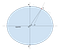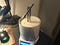# Calculating Azimuth, Distance, and Altitude from a Pair of GPS Locations in JavaScript

## An algorithm for finding your drone or aiming a satellite dish, with sample code in JavaScript

Suppose you are standing somewhere on the Earth, and there is another location on Earth that you would like to face, approach, or aim a telescope toward. If you have GPS coordinates — latitude, longitude, and elevation — for both locations, I will show you an algorithm for calculating two direction angles that aim your starting point to that target point. In addition, the algorithm calculates the distance between the points. I also provide a web-based calculator that implements this algorithm, using open-source JavaScript code.

## The Input

• Latitude: how many degrees north or south of the Earth’s equator the point is. Positive values are north of the equator, and negative values are south of the equator.
• Longitude: how many degrees east (positive) or west (negative) the point is from the prime meridian in Greenwich, England.
• Elevation: the point’s height above (positive) or below (negative) mean sea level, expressed in meters.

## The Output

• Azimuth: The compass direction of Point B as seen from Point A. This is a number from 0 to 360 degrees. The value 0 indicates north, 90 is east, 180 is south, and 270 is west.
• Altitude: This is the angle above (positive) or below (negative) the horizon of Point B as seen by an observer at Point A. This is not to be confused with the elevation input defined above.
• Distance: The straight-line distance (not the distance around the Earth) from Point A to Point B, expressed in kilometers.

## How It WorksGeocentric latitude θ is not the same as geodetic latitude φ on the oblate Earth.

Here, X is a point on the Earth’s surface, N is the north pole, S is the south pole, and C is the center of the Earth. The equator is represented by the horizontal line passing through C. Imagine you are standing at the point X. The dotted line tangent to the Earth there indicates your local horizon.

Perpendicular to your horizon is another dotted line that passes into the Earth. Where that dotted line touches the plane of the Earth’s equator, you see an angle marked φ (Greek letter phi). This angle is called the geodetic latitude. This is the kind of latitude reported by GPS.

Indeed, geodetic latitude is the classic kind of latitude used since ancient times. Roughly speaking, it indicates a latitude measured by astronomy: it is the angle of the North Celestial Pole (a point in the sky near the pole star Polaris) above your local horizon. If you are standing on the Earth’s north pole, Polaris will be straight up, or 90 degrees above the horizon. Likewise, if you are standing on the equator, Polaris will be on the horizon, or 0 degrees above the horizon. Thus geodetic latitude can be measured directly by any sailor with a clear nighttime sky.

However, to calculate the Cartesian coordinates of X with respect to the center C of the Earth, the calculator needs geocentric latitude, indicated by θ (Greek letter theta) in the diagram. This is the angle between the equator and X as seen from C. The conversion is based on the World Geodetic System standard WGS 84. In the calculator this is implemented in the function `GeocentricLatitude`, shown here:

Likewise, the distance from the center of the Earth to a given point on its surface is variable. The function `EarthRadiusInMeters` calculates this distance as a function of geodetic latitude, also using WGS 84:

As an interesting aside, geocentric and geodetic latitudes are the same when you are on the equator: both are 0 degrees. Both kinds of latitude are also the same if you are on either of the Earth’s poles: +90 or -90 degrees. But they disagree by different amounts at other locations on the Earth.

The calculator uses the function `LocationToPoint` to calculate the Cartesian coordinates of a point given its geodetic latitude, longitude, and elevation. This function also calculates the normal vector at the given point, meaning the upward direction perpendicular to the observer’s horizon, to correctly adjust for elevation above mean sea level. The resulting vector components are all expressed in meters.

To complete the calculations, the algorithm uses a trick. It rotates the coordinate system so that the observation point (Point A) is at a pretend equator and prime meridian. In other words, imagine rotating a globe you are holding so that your observation site is now on the equator and directly facing you. The coordinates now are ideally situated for calculating azimuth and altitude angles as seen by the observer at Point A:

• The x-axis points straight up (out from the center of the globe).
• The y-axis points due east.
• The z-axis points due north.

Here is the rotation function `RotateGlobe`:

After `RotateGlobe` rotates the coordinate system, you can draw a line from Point A on the rotated globe toward Point B on that same rotated globe, through three-dimensional space. This line will usually pass through the Earth, assuming both A and B are on or near the Earth’s surface. Using a combination of trigonometry functions and vector dot products, the calculator determines the azimuth angle and altitude angles. It uses the Pythagorean formula to determine the straight-line distance between the points.

All of the code is available in the links below.

## Example #1: International Space Station PointerISS Pointer project by A. Busch, R. Pick, M. Schmidt, J. Steinberg; Bonn-Rhein-Sieg University of Applied Sciences, Germany.

## Other Examples

• A man who races sailboats uses my tool to help plan routes through the required waypoints of a race.
• A microwave dish technician used my tool to help aim microwave relays from one tower to another.
• A satellite dish installer said my tool helped aim dishes to specific satellites. I added the “geostationary satellite” checkbox based on his suggestion, so he didn’t have to enter the elevation number manually.
• I originally wrote this tool so I could experiment with magnetic loop antennas for AM receivers I built. These antennas are highly sensitive to the direction of the broadcast tower. By rotating the receiver, I can tell which direction the signal is coming from. I could correlate this with known GPS coordinates for the broadcast towers to verify I was really receiving from the tower I thought I was.

Feel free to comment here to share how you use this code! I’m always interested in the creative things people do.

Written by

## JavaScript in Plain English

#### Learn the web's most important programming language.

Welcome to a place where words matter. On Medium, smart voices and original ideas take center stage - with no ads in sight. Watch
Follow all the topics you care about, and we’ll deliver the best stories for you to your homepage and inbox. Explore
Get unlimited access to the best stories on Medium — and support writers while you’re at it. Just \$5/month. Upgrade